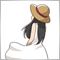# Coding Help : Custom Indicator Function in EA29

Dear coders,

this is part of my EA and I put BBand Stop indicator into the EA by using function, I don't want using iCustom function to get Buffer value.

The problems is final array print in journal shows wrong value, I appreciated if you check it what is the problem.

for your information journal just shows BBandUpLineArray value and all array shows wrong values.

```extern int                   BBandsLength        = 9; // Bollinger Bands Period
extern int                   BBandsDeviation     = 4; // Deviation
extern ENUM_MA_METHOD        BBandsMaMethod      = 0; // MA Method
extern ENUM_APPLIED_PRICE    BBandsPrice         = 0; // Price

double   BBandUpLineArray, BBandDnLineArray;

void OnTick()
{
int limit = BBandsLength;

FunBBandsStop(limit, BBandsLength, BBandsDeviation, BBandsMaMethod, BBandsPrice, BBandUpLineArray, BBandDnLineArray);

for (int i = 0; i < limit; i++)
{
if (BBandDnLineArray[i] > 0)
Print("BBandDnLineArray : ", NormalizeDouble(BBandDnLineArray[i], Digits), "   Array : ", i);
if (BBandUpLineArray[i] > 0)
Print("BBandUpLineArray : ", NormalizeDouble(BBandUpLineArray[i], Digits), "   Array : ", i);
}
}

//+------------------------------------------------------------------+
void FunBBandsStop(int limit, int BBandslength, int BBandsdeviation, int BBandsmaMethod, int BBandsprice, double& UpLineBuffer[], double& DnLineBuffer[])
{
double smax[];
double smin[];
double trend[];
ArrayResize(smax, 10);
ArrayResize(smin, 10);
ArrayResize(trend, 10);
ArrayResize(UpLineBuffer, 10);
ArrayResize(DnLineBuffer, 10);
for (int i = 0; i < limit; i++)
{
double StdDev  = iStdDev(NULL, 0, BBandslength, 0, MODE_SMA, BBandsprice, i);
double ma      = iMA(NULL, 0, BBandslength, 0, BBandsmaMethod, BBandsprice, i);
smax[i] = ma + (StdDev * BBandsdeviation);
smin[i] = ma - (StdDev * BBandsdeviation);
trend[i]   = trend[i + 1];
if (Close[i] > smax[i + 1]) trend[i] = 1;
if (Close[i] < smin[i + 1]) trend[i] = -1;
if (trend[i] > 0 && smin[i] < smin[i + 1]) smin[i] = smin[i + 1];
if (trend[i] < 0 && smax[i] > smax[i + 1]) smax[i] = smax[i + 1];
if (trend[i] == 1) UpLineBuffer[i] = smin[i];
if (trend[i] == -1) DnLineBuffer[i] = smax[i];
}
return;
}```

Files:504

`for (int i = limit; i >= 0; i--)`

Because when calculating from 0 to limit, the value of i + 1 has not yet been determined.29